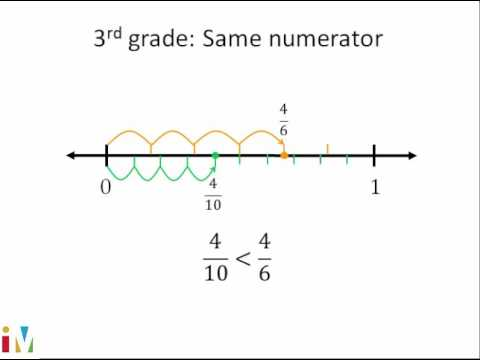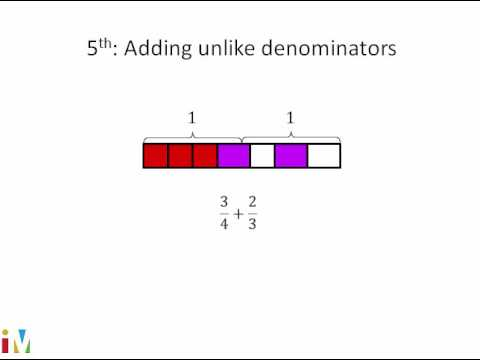Video

# Fraction word problem: piano (Full video)

Description: Sal solves a fraction word problem about practicing piano. The fractions have common denominators. So if this represents an entire hour, so let's divide it into fourths. Now, let's make it into four equal sections. So he needs to practice 3/4 of an hour.

### Other videos you might be interested in### Comparing Fractions

#### Illustrative Mathematics